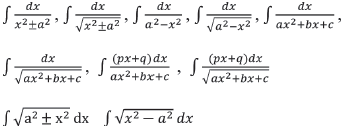Saturday 04th December 2021
 All India Engineering / Architecture Entrance Examination (AIEEE) - 2013 CBSE Guess > AIEEE > AIEEE Syllabus > AIEEE 2013 Mathematics Syllabus AIEEE 2013 Mathematics Syllabus UNIT 1: SETS, RELATIONS AND FUNCTIONS: Sets and their representation; Union, intersection and complement of sets and their algebraic properties; Power set; Relation, Types of relations, equivalence relations, functions;. one-one, into and onto functions, composition of functions. UNIT 2: COMPLEX NUMBERS AND QUADRATIC EQUATIONS: Complex numbers as ordered pairs of reals, Representation of complex numbers in the form a+ib and their representation in a plane, Argand diagram, algebra of complex numbers, modulus and argument (or amplitude) of a complex number, square root of a complex number, triangle inequality, Quadratic equations in real and complex number system and their solutions. Relation between roots and co-efficients, nature of roots, formation of quadratic equations with given roots. UNIT 3: MATRICES AND DETERMINANTS: Matrices, algebra of matrices, types of matrices, determinants and matrices of order two and three. Properties of determinants, evaluation of determinants, area of triangles using determinants. Adjoint and evaluation of inverse of a square matrix using determinants and elementary transformations, Test of consistency and solution of simultaneous linear equations in two or three variables using determinants and matrices. UNIT 4: PERMUTATIONS AND COMBINATIONS: Fundamental principle of counting, permutation as an arrangement and combination as selection, Meaning of P (n,r) and C (n,r), simple applications. UNIT 5: MATHEMATICAL INDUCTION: Principle of Mathematical Induction and its simple applications. UNIT 6: BINOMIAL THEOREM AND ITS SIMPLE APPLICATIONS: Binomial theorem for a positive integral index, general term and middle term, properties of Binomial coefficients and simple applications. UNIT 7: SEQUENCES AND SERIES: Arithmetic and Geometric progressions, insertion of arithmetic, geometric means between two given numbers. Relation between A.M. and G.M. Sum upto n terms of special series: Sn, Sn2, Sn3. Arithmetico - Geometric progression. UNIT 8: LIMIT, CONTINUITY AND DIFFERENTIABILITY: Real - valued functions, algebra of functions, polynomials, rational, trigonometric, logarithmic and exponential functions, inverse functions. Graphs of simple functions. Limits, continuity and differentiability. Differentiation of the sum, difference, product and quotient of two functions. Differentiation of trigonometric, inverse trigonometric, logarithmic, exponential, composite and implicit functions; derivatives of order upto two. Rolle’s and Lagrange’s Mean Value Theorems. Applications of derivatives: Rate of change of quantities, monotonic - increasing and decreasing functions, Maxima and minima of functions of one variable, tangents and normals. UNIT 9: INTEGRAL CALCULUS: Integral as an anti - derivative. Fundamental integrals involving algebraic, trigonometric, exponential and logarithmic functions. Integration by substitution, by parts and by partial fractions. Integration using trigonometric identities. Evaluation of simple integrals of the typeIntegral as limit of a sum. Fundamental Theorem of Calculus. Properties of definite integrals. Evaluation of definite integrals, determining areas of the regions bounded by simple curves in standard form. UNIT 10: Differential Equations: Ordinary differential equations, their order and degree. Formation of differential equations. Solution of differential equations by the method of separation of variables, solution of homogeneous and linear differential equations of the type: dy -- + p (x) y = q (x) dx UNIT 11: CO-ORDINATE GEOMETRY: Cartesian system of rectangular co-ordinates in a plane, distance formula, section formula, locus and its equation, translation of axes, slope of a line, parallel and perpendicular lines, intercepts of a line on the coordinate axes. Straight lines Various forms of equations of a line, intersection of lines, angles between two lines, conditions for concurrence of three lines, distance of a point from a line, equations of internal and external bisectors of angles between two lines, coordinates of centroid, orthocentre and circumcentre of a triangle, equation of family of lines passing through the point of intersection of two lines. Circles, conic sections Standard form of equation of a circle, general form of the equation of a circle, its radius and centre, equation of a circle when the end points of a diameter are given, points of intersection of a line and a circle with the centre at the origin and condition for a line to be tangent to a circle, equation of the tangent. Sections of cones, equations of conic sections (parabola, ellipse and hyperbola) in standard forms, condition for y = mx + c to be a tangent and point (s) of tangency. UNIT 12: Three Dimensional Geometry: Coordinates of a point in space, distance between two points, section formula, direction ratios and direction cosines, angle between two intersecting lines. Skew lines, the shortest distance between them and its equation. Equations of a line and a plane in different forms, intersection of a line and a plane, coplanar lines. UNIT 13: Vector Algebra: Vectors and scalars, addition of vectors, components of a vector in two dimensions and three dimensional space, scalar and vector products, scalar and vector triple product. UNIT 14: STATISTICS AND PROBABILITY: Measures of Dispersion: Calculation of mean, median, mode of grouped and ungrouped data. Calculation of standard deviation, variance and mean deviation for grouped and ungrouped data. Probability: Probability of an event, addition and multiplication theorems of probability, Baye’s theorem, probability distribution of a random variate, Bernoulli trials and Binomial distribution. UNIT 15: Trigonometry: Trigonometrical identities and equations. Trigonometrical functions. Inverse trigonometrical functions and their properties. Heights and Distances. UNIT 16: MATHEMATICAL REASONING: Statements, logical operations and, or, implies, implied by, if and only if. Understanding of tautology, contradiction, converse and contrapositive. AIEEE 2013 Syllabus